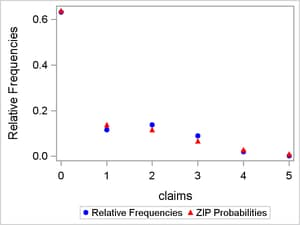# SAS/STAT Examples

## High-Performance Variable Selection for Generalized Linear Models: PROC HPGENSELECT

Contents | SAS Program | PDF

## Overview

The HPGENSELECT procedure, available in SAS/STAT 12.3 (which runs on Base SAS 9.4), performs model selection for generalized linear models (GLMs). It fits models for standard distributions in the exponential family, such as the normal, Poisson, and Tweedie distributions. In addition, PROC HPGENSELECT fits multinomial models for ordinal and nominal responses, and it fits zero-inflated Poisson and negative binomial models for count data. For all these models, the HPGENSELECT procedure provides forward, backward, and stepwise variable selection and includes Akaike’s information criterion (AIC), a small-sample bias-corrected version of Akaike’s information criterion (AICC), and the Schwarz Bayesian criterion (SBC) as selection criteria. PROC HPGENSELECT enables you to run in distributed mode on a cluster of machines that distribute the data and the computations or in single-machine mode on the server where SAS is installed.

## Analysis

Many of the probability distributions that the HPGENSELECT procedure fits are members of an exponential family of distributions, which have probability distributions that are expressed as follows for some functionsandthat determine the specific distribution: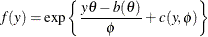For fixed, this is a one-parameter exponential family of distributions. The response variable can be discrete or continuous, so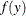represents either a probability mass function or a probability density function. A more useful parameterization of generalized linear models is by the mean and variance of the distribution: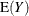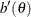In generalized linear models, the mean of the response distribution is related to linear regression parameters through a link function,for the ith observation, where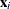is a fixed known vector of explanatory variables andis a vector of regression parameters. The HPGENSELECT procedure parameterizes models in terms of the regression parametersand either the dispersion parameteror a parameter that is related to, depending on the model. For exponential family models, the distribution variance is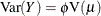, where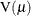is a variance function that depends only on.

The zero-inflated models and the multinomial models are not exponential family models, but they are closely related models that are useful and are included in the HPGENSELECT procedure.

### Zero-Inflated Models

Count data that have an incidence of zeros greater than expected for the underlying probability distribution of counts can be modeled by using a zero-inflated distribution. In PROC HPGENSELECT, the underlying distribution can be either Poisson or negative binomial. The population is considered to consist of two types of individuals. The first type gives Poisson or negative binomial distributed counts, which might contain zeros. The second type always gives a zero count. Supposeis the underlying distribution mean andis the probability of an individual being of the second type. The parameter, which is called the zero-inflation probability, is the probability of zero counts in excess of the frequency that the underlying distribution predicts.

The probability distribution of a zero-inflated Poisson random variable Y is given byThe probability distribution of a zero-inflated negative binomial random variable Y is given bywhere k is the negative binomial dispersion parameter.

You can model the parametersandin PROC HPGENSELECT by using the regression models,where h is one of the binary link functions: logit, probit, or complementary log-log. You usually use the log link function for g when you are fitting a Poisson or a negative binomial model.

The mean and variance of Y for the zero-inflated Poisson are given by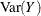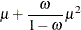The mean and variance of Y for the zero-inflated negative binomial are given by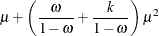### Multinomial Models

Multinomial models apply to cases where an observation can fall into one of k categories. Binary data occur in the special case where k = 2. If there are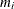observations in a subpopulation i, then the probability distribution of the number that falls into the k categories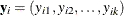can be modeled by the multinomial distribution, where. The multinomial model is an ordinal model if the categories have a natural order.

If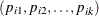are the category probabilities, the cumulative category probabilities are modeled by using the same link functions that are used for binomial data. Suppose that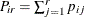,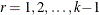, are the cumulative category probabilities. The ordinal model is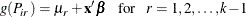where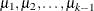are intercept terms that depend only on the categories andis a vector of covariates that does not include an intercept term. The link function g can be specified as a logit, probit, log-log, or complementary log-log function.

### Model Selection

The HPGENSELECT procedure supports three methods of effect selection: forward selection, backward elimination, and stepwise selection.

In forward selection, the model-fitting process begins with only the intercept and then sequentially adds the effect that most improves the fit. The process terminates when adding an effect produces no significant improvement. The statistic that determines whether to add an effect is the significance level of a hypothesis test that indicates an effect’s potential contribution to the model. At each step, the effect that is most significant is added. The process stops when the significance level for adding any effect is greater than some specified entry significance level.

Backward elimination starts from the full model, which includes all independent effects. Then effects are deleted one by one until a stopping condition is satisfied. At each step, the effect that makes the smallest contribution to the model is deleted. The significance level of an effect determines whether to drop that effect. At any step, the least significant predictor is dropped, and the process continues until all effects that remain in the model are significant at a specified stay significance level.

Stepwise selection is a modification of forward selection in which effects already in the model do not necessarily stay there. In the HPGENSELECT procedure’s implementation of stepwise selection, the same entry and removal significance levels for forward selection and backward elimination are used to assess contributions of effects as they are added to or removed from a model. If, at a step of the selection process, any effects in the model are not significant, then the least significant of these effects is removed from the model and the algorithm proceeds to the next step. This ensures that no effect can be added to a model while an effect currently in the model is not deemed significant. Only after all necessary deletions have been made can another effect be added to the model. In this case the effect whose addition is the most significant is added to the model, and the algorithm proceeds to the next step. The stepwise process ends when none of the effects outside the model are significant and every effect in the model is significant. In some cases, neither of these two stopping conditions is met and the sequence of models cycles. In these cases, the stepwise method terminates at the end of the sequence.

## Example: Modeling Automobile Insurance Claims

Frequency models are commonly used in the insurance industry to predict how often claims are made. This example uses a sample of real automobile insurance policy data to model the number of claims. The following DATA step reads the data, and Table 1 describes the variables in the data set Claim_History:


data claim_history;
input ID $1-10 Kids_Drive 11 Birth$ 15-25  Age 27-30
Home_Kids 31 YOJ 34-37 Income 38-48 Parent1 $49-52 Home_Value 53-63 MStatus$ 64-67 Gender $68 #2 Education$ 1-14 Occupation $15-27 Travel_Time 28-34 Car_Use$ 35-45 Bluebook 46-55 TIF 56 Car_Type $60-72 #3 Red_Car$ 1-3 OldClaim 5-13 Claims 14-18 Revoked $19-23 Mvr_Pts 24-26 Clm_Amt 27-35 Car_Age 36-39 Claim_Flag 40 Urbanicity$ 43-48;
datalines;
77382913  0   11/22/1964  34  0  10  62977.82   No  0.00       No  F

... more lines ...

121441578 0   7/1/1964    35  0  11  43111.84   No  0.00       No  M
High School   Blue Collar  51.00  Commercial 27330.00  10  Panel Truck
Yes 0.00     0    No   0  0.00     8   0  Rural
;


Table 1: Claim_History Data Set

Variable Name

Description

ID

Policy identification number

Kids_Drive

Number of driving children

Birth

Date of birth of insured

Age

Age of insured

Home_Kids

Number of children at home

YOJ

Years on job

Income

Income of insured

Parent1

Single parent

Home_Value

Value of home

MStatus

Marital status

Gender

Gender of insured

Education

Maximum education level of insured

Occupation

Occupation of insured

Travel_Time

Distance to work

Car_Use

Vehicle use

Bluebook

Value of vehicle

TIF

Time in force

Car_Type

Type of vehicle

Red_Car

A red car

OldClaim

Total dollar value of claims in past five years

Claims

Number of claims in past five years

Revoked

License revoked in past seven years

Mvr_Pts

Motor vehicle record points

Clm_Amt

Claim amount

Car_Age

Age of vehicle

Claim_Flag

Claim indicator

Urbanicity

Home/Work area

You can use PROC FREQ as follows to generate a histogram of the response variable Claims for a visual inspection of its marginal distribution:


ods graphics on;
proc freq data=claim_history;
table claims / plots(only)=freqplot(scale=percent);
run;


Figure 1 shows that the marginal distribution of Claims resembles a Poisson distribution that has excess zeros, suggesting that a zero-inflated Poisson (ZIP) model might be appropriate.

Figure 1: Distribution of Claims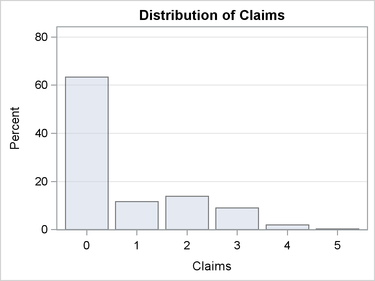The following SAS statements fit a zero-inflated Poisson (ZIP) model and use forward selection to find the best subset of effects for both the conditional mean of the Poisson distribution and the zero-inflation probability.

The CLASS statement specifies that the variables Education, Gender, Car_Type, Car_Use, MStatus, Occupation, Parent1, Red_Car, Revoked, and Urbanicity are categorical variables.

The MODEL statement specifies that the response variable has a zero-inflated Poisson distribution with a log link function.

The ZEROMODEL statement requests that a probit link function be used for the zero-inflation probability equation.

The SELECTION statement requests that forward selection be used and that the final model be chosen based on the AICC criterion. The DETAILS=SUMMARY option requests that only a summary of the selection process be displayed rather than the details from each step of the selection process.

The ID statement requests that the variable Claims be included in the output data set.

The OUTPUT statement requests that the selected model’s prediction and the estimate of the zero-inflation probability for each observation be saved to the SAS data set Zip.

The ODS OUTPUT statements requests that the selected model’s Fit Statistics table be saved to the SAS data set Fit, the Number of Observations table be saved to the SAS data set Nobs, and the Dimensions table be saved to the SAS data set Dimensions.


proc hpgenselect data=claim_history;
class education gender car_type car_use mstatus occupation parent1
red_car revoked urbanicity;
model claims = education gender car_type car_use mstatus occupation
parent1 red_car revoked urbanicity bluebook age
car_age home_kids home_value income kids_drive mvr_pts tif
zeromodel education gender car_type car_use mstatus occupation parent1
red_car revoked urbanicity bluebook age car_age home_kids
home_value income kids_drive mvr_pts tif travel_time yoj / link=probit;
selection method=forward(choose=aicc) details=summary;
id claims;
output out=zip pred=pred pzero=pzero;
ods output fitstatistics=fit nobs=nobs dimensions=dimensions;
run;


The Performance Information table in Output 1 shows that the procedure executed in single-machine mode (that is, on the server where SAS is installed). When high-performance procedures run in single-machine mode, they use concurrently scheduled threads. In this case, four threads were used.

The Model Information table reports that a zero-inflated Poisson model was fit with a log link function for the mean equation and a probit link function for the zero-inflation probability equation.

Output 1: Performance and Model Information

The HPGENSELECT Procedure

Performance Information
Execution Mode Single-Machine

Model Information
Data Source WORK.CLAIM_HISTORY
Response Variable Claims
Class Parameterization GLM
Distribution Zero-Inflated Poisson
Optimization Technique Newton-Raphson with Ridging

 Number of Observations Read 2500 2500

Output 2 lists the 10 class variables that are specified in the CLASS statement, along with the number of levels and the values for each variable.

Output 2: Class Level Information

Class Level Information
Class Levels Values
Education 5 < High School Bachelors High School Masters PhD
Gender 2 F M
Car_Type 6 Minivan Panel Truck Pickup SUV Sports Car Van
Car_Use 2 Commercial Private
MStatus 2 No Yes
Occupation 8 Blue Collar Clerical Doctor Home Maker Lawyer Manager Professional Student
Parent1 2 No Yes
Red_Car 2 No Yes
Revoked 2 No Yes
Urbanicity 2 Rural Urban

Output 3 displays a summary of the selection process.

The Selection Information table reports that forward selection was used, the selection and stopping criteria are the significance level of each individual effect, and the entry significance level is the default value of 0.05.

The Selection Summary table reports the variables that are added at each step of the selection process. Because the ZIP model is a mixture model and has two equations, the variables that are selected for the zero-inflation probability equation are distinguished from the variables that are selected for the mean equation by having _Zero appended to their name. The summary shows that four variables plus an intercept were selected for the mean equation and that eight variables plus an intercept were selected for the zero-inflation probability equation.

The Dimensions table reports that 14 effects are included in the final model and 18 parameters are estimated.

Output 3: Summary of the Selection Process

Selection Information
Selection Method Forward
Select Criterion Significance Level
Stop Criterion Significance Level
Choose Criterion AICC
Effect Hierarchy Enforced None
Entry Significance Level (SLE) 0.05
Stop Horizon 1

The HPGENSELECT Procedure

Selection Summary
Step Effect
Entered
Number
Effects In
AICC p Value
0 Intercept 1
Intercept_Zero 2 5686.6821 .
1 Mvr_Pts_Zero 3 5140.5067 <.0001
2 Urbanicity_Zero 4 4979.1354 <.0001
3 Income_Zero 5 4954.3377 <.0001
4 Car_Use_Zero 6 4937.4125 <.0001
5 Revoked_Zero 7 4926.9092 0.0005
6 Travel_Time_Zero 8 4920.1708 0.0031
7 Car_Type_Zero 9 4914.0826 0.0064
8 Home_Value_Zero 10 4908.7648 0.0070
9 Red_Car 11 4904.6657 0.0126
10 MStatus 12 4901.7134 0.0254
11 Travel_Time 13 4899.3634 0.0358
12 Mvr_Pts 14 4897.1158* 0.0383

 * Optimal Value of Criterion

 Selection stopped because no candidate for entry is significant at the 0.05 level.

 The model at step 12 is selected where AICC is 4897.116.

Selected Effects: Intercept MStatus Red_Car Mvr_Pts Travel_Time Intercept_Zero Car_Type_Zero Car_Use_Zero Revoked_Zero Urbanicity_Zero Home_Value_Zero Income_Zero Mvr_Pts_Zero Travel_Time_Zero

Dimensions
Number of Effects 14
Number of Parameters 18
Columns in X 24

Output 4 displays the estimates of the model parameters. The Parameter Estimates table displays the estimates of the mean equation’s parameters, and the Zero-Inflation Parameter Estimates table displays the estimates of the zero-inflation probability equation’s parameters. The selected variables for the mean equation are MStatus, Red_Car, Mvr_Pts, and Travel_Time. The selected variables for the zero-inflation probability equation are Car_Type, Car_Use, Revoked, Urbanicity, Home_Value, Income, Mvr_Pts, and Travel_Time.

Output 4: Parameter Estimates

Parameter Estimates
Parameter DF Estimate Standard
Error
Chi-Square Pr > ChiSq
Intercept 1 0.393835 0.087850 20.0977 <.0001
MStatus No 1 0.111187 0.052546 4.4775 0.0343
MStatus Yes 0 0 . . .
Red_Car No 1 -0.142290 0.056806 6.2743 0.0123
Red_Car Yes 0 0 . . .
Mvr_Pts 1 0.021451 0.010358 4.2888 0.0384
Travel_Time 1 0.003631 0.001707 4.5255 0.0334

Zero-Inflation Parameter Estimates
Parameter DF Estimate Standard
Error
Chi-Square Pr > ChiSq
Intercept_Zero 1 0.281308 0.199009 1.9981 0.1575
Car_Type_Zero Minivan 1 0.095028 0.148198 0.4112 0.5214
Car_Type_Zero Panel Truck 1 -0.034481 0.200538 0.0296 0.8635
Car_Type_Zero Pickup 1 -0.072832 0.157468 0.2139 0.6437
Car_Type_Zero SUV 1 -0.297481 0.151435 3.8589 0.0495
Car_Type_Zero Sports Car 1 -0.297123 0.179088 2.7526 0.0971
Car_Type_Zero Van 0 0 . . .
Car_Use_Zero Commercial 1 -0.367408 0.091243 16.2144 <.0001
Car_Use_Zero Private 0 0 . . .
Revoked_Zero No 1 0.380971 0.111722 11.6281 0.0006
Revoked_Zero Yes 0 0 . . .
Urbanicity_Zero Rural 1 1.390559 0.108296 164.8738 <.0001
Urbanicity_Zero Urban 0 0 . . .
Home_Value_Zero 1 0.000000814 0.000000362 5.0499 0.0246
Income_Zero 1 0.000002556 0.000001024 6.2366 0.0125
Mvr_Pts_Zero 1 -0.363820 0.023392 241.9059 <.0001
Travel_Time_Zero 1 -0.005264 0.002499 4.4386 0.0351

 Convergence criterion (GCONV=1E-8) satisfied.

Output 5 displays the fit statistics for the final model.

Output 5: Fit Statistics

Fit Statistics
-2 Log Likelihood 4860.84011
AIC (smaller is better) 4896.84011
AICC (smaller is better) 4897.11580
BIC (smaller is better) 5001.67293
Pearson Chi-Square 2398.50471
Pearson Chi-Square/DF 0.96636

Most of the criteria are useful only for comparing the model fit among given alternative models. However, the Pearson statistic can be used to determine whether there is evidence of overdispersion or underdispersion. If the model is correctly specified and there is no overdispersion or underdispersion, the Pearson chi-square statistic divided by the degrees of freedom has an expected value of 1. The obvious question is whether the observed value of 0.96636 is significantly less than 1 and thus indicates underdispersion. The Pearson statistic for a zero-inflated Poisson model has a limiting chi-square distribution under certain regularity conditions, with degrees of freedom equal to the number of observations minus the number of estimated parameters. A formal one-sided test for underdispersion is performed by computing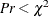, the probability of observing a smaller value of the statistic.

The following SAS statements compute the p-value for the test:


data _null_;
set nobs(where=(label="Number of Observations Used"));
call symput('n',NobsUsed);
run;

data _null_;
set dimensions(where=(description="Number of Parameters"));
call symput('parms',value);
run;

data fit;
set fit(where=(label="Pearson Chi-Square"));
format pvalue pvalue6.4;
df=%eval(&n) - %eval(&parms);
pvalue=probchi(value,df);
label pvalue="Pr < ChiSq";
run;


proc print data=fit noobs label;
var label value df pvalue;
run;


Output 6 displays the test result. The p-value for the one-sided test for underdispersion is 0.12, so you fail to reject the null hypothesis of no underdispersion at the most commonly used confidence levels.

Output 6: Test for Overdispersion

Description Value df Pr < ChiSq
Pearson Chi-Square 2398.50471 2482 0.1172

A common method of assessing the goodness of fit of a model is to compare the observed relative frequencies of the various counts to the maximum likelihood estimates of their respective probabilities. The following SAS statements demonstrate one method of computing the estimated probabilities and generating two comparative plots.

The first step is to observe the value of the largest count and save it as a macro variable:


proc means data=zip(where=(~missing(pred))) noprint;
var claims;
output out=maxcount max=max;
run;


data _null_;
set maxcount;
call symput('max',max);
run;

%let max=%sysfunc(strip(&max));


Next, you use the model predictions and the estimated zero-inflation probabilities that are stored in the output data set Zip to compute the conditional probabilities. These are the variables ep0ep&max in the following DATA step. You also generate an indicator variable for each count,, where each observation is assigned a value of 1 if countis observed, and 0 otherwise. These are the variables c0c&max.


data zip(drop= i);
set zip(where=(~missing(pred)));
lambda=pred/(1-pzero);
array ep{0:&max} ep0-ep&max;
array c{0:&max} c0-c&max;
do i = 0 to &max;
if i=0 then ep{i}= pzero + (1-pzero)*pdf('POISSON',i,lambda);
else        ep{i}=         (1-pzero)*pdf('POISSON',i,lambda);
c{i}=ifn(claims=i,1,0);
end;
run;


Now you can use PROC MEANS to compute the means of the variables ep0,, ep&max and c0,, c&max. The means of ep0,, ep&max are the maximum likelihood estimates of. The means of c0,, c&max are the observed relative frequencies.


proc means data=zip noprint;
var ep0 - ep&max c0-c&max;
output out=ep(drop=_TYPE_ _FREQ_) mean(ep0-ep&max)=ep0-ep&max;
output out=p(drop=_TYPE_ _FREQ_) mean(c0-c&max)=p0-p&max;
run;


The output data sets from PROC MEANS are in what is commonly referred to as wide form. That is, there is one observation for each variable. In order to generate comparative plots, the data need to be in what is referred to as long form. Ultimately, you need four variables: one whose observations are an index of the values of the counts, a second whose observations are the observed relative frequencies, a third whose observations contain the ZIP model estimates of the probabilities, and a fourth whose observations contain the difference between the observed relative frequencies and the estimated probabilities.

The following SAS statements transpose the two output data sets so that they are in long form. Then, the two data sets are merged, and the variables that index the count values and record the difference between the observed relative frequencies and the estimated probabilities are generated.


proc transpose data=ep out=ep(rename=(col1=zip) drop=_NAME_);
run;

proc transpose data=p out=p(rename=(col1=p) drop=_NAME_);
run;


data zipprob;
merge ep p;
zipdiff=p-zip;
claims=_N_ -1;
label zip='ZIP Probabilities'
p='Relative Frequencies'
zipdiff='Observed minus Predicted';
run;


Now you can use the SGPLOT procedure to produce the comparative plots:


proc sgplot data=zipprob;
scatter x=claims y=p /
markerattrs=(symbol=CircleFilled size=5px color=blue);
scatter x=claims y=zip /
markerattrs=(symbol=TriangleFilled size=5px color=red);
xaxis type=discrete;
run;


Figure 2 shows that the ZIP model that has the selected effects captures the shape of the distribution of the relative frequencies and accounts for the excess zeros quite well.

Figure 2: Comparison of ZIP Probabilities to Observed Relative Frequencies

 ZIP Probabilities versus Relative Frequencies Observed Relative Frequencies Minus ZIP Probabilities